03 Ideal ADC, DNL and INL

Prof. Dr. Jörg Vollrath

Previous: 02 LTSPICE

Video 3. lecture

 Video is not visible, most likely your browser does not support HTML5 video Länge: 01:06:27 0:0:0 Start 0:1:22 ADC Converter metric 0:3:23 ADC transfer characteristic 0:4:40 First transition half LSB 0:6:35 2^n codes, 2^n-1 transitions, 2^n-2 steps 0:8:17 Transition table 0:9:15 Offset and gain error of ADC 0:11:39 Differential non linearity DNL 0:14:37 3-bit, 8 codes, transitions 7, steps 6 0:17:16 DNL calculation 0:17:48 Sum DNL is zero 0:18:50 Integral non linearity INL 0:19:44 measurement of INL and DNL 0:21:32 Histogram test 0:26:30 INL sum of DNL 0:28:25 ADC Error simulator 0:29:11 Simulator ideal ADC ramp result 0:31:55 Introducing an distortion error 0:32:34 Transfer curve INl, DNL 0:35:29 Exam WS2010 Problem 1 0:36:50 LSB =(VMAX-VMIN)/(2^n-2) = (1.21-0.09)/14 V = 0.08 V 0:39:3 Math Notepad 0:39:45 Offset error 0.09-0.08/2 = 0.05 V 0:42:50 INL and DNL 0:44:13 INL(0100) = 0.525 0:46:0 DNL(0100) = 0.2 0:48:22 WS2011 Problem 1 Histogram 0:49:10 Don't use first and last occurences 0:50:0 navg = 15 0:50:59 DNL(1) = -0.2 0:52:0 DNL(2) = 0 0:53:0 INL(1) = -0.2 0:55:0 Resolution of histogram test 1/15 0:56:45 Simulator 0:57:27 INL, DNL simulation with noise 1:0:0 8-bit simulation 1:2:50 small distortion 1:4:40 Sine input for histogram 1:7:40 Number of samples for sine histogram 1:10:43 Characterization system 1:14:15 WS2011 Problem 1 DAC 1:15:30 Excel data 1:16:10 First and last ideal voltage 1:16:30 LSB calculation 1:17:28 Ideal output voltages 1:18:24 LSB accuracy 1:19:37 DNL calculation 1:20:40 INL calculation 1:22:12 Plotting transfer curve 1:24:2 Plotting INL, DNL

Review and Overview

Review

• Properties of digital signals
• Applications of data converters
• DAC metric: N, D, Vmax
• LTSPICE basic operation:
• R,L,C,V,M,Q,X components
• simulation: .op .dc .tran
• LTSPICE data converter simulation

Overview

• ADC metric: N, D, Vmax
• Static errors: Offset, gain, INL, DNL
• Histogram test with ramp and sine signal

Baker:
Holberg:

 N: Number of Bits D0..DN-1: Binary weighted data lines Vin: Positiv input voltage Vmax: Maximum input voltage VFS: Full scale voltage Vref: Reference voltage Δ = LSB: minimum resolvable input For small N there is a significant difference LSB or Δ between Vmax, VFS and Vref. In general $V_{max} = V_{FS} = V_{ref} - LSB$ (Baker). For large N, LSB gets small and Vmax = VFS ≈ Vref.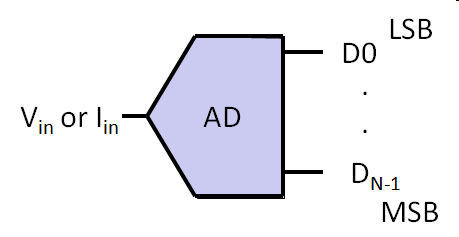Ideal analog-to-digital converter An analog voltage or current is transfered into a digital output. Input range is positiv. Uniform, binary digital encoding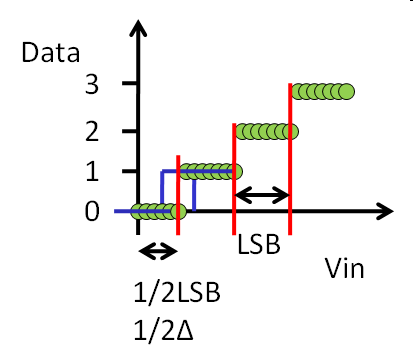Transition depends on measurement accuracy and step size.
Vref = 1V
N = 2
LSB = 0.25V

Upper voltage limit (transition voltage) for a digital output code:
 Vin [V] D1 D0 ideal voltage VT(00/01) 0.125 0 0 1/2 LSB VT(01/10) 0.375 0 1 3/2 LSB VT(10/11) 0.625 1 0 5/2 LSB -- 1 1

N: Number of bits
Vref: Reference voltage

Example: N = 12, Vref = 4 V

$LSB_{rel} = \frac{1}{2^{12}} = \frac{1}{4096} = 0.00024414 = 244 ppm$

$LSB_{abs} = \frac{4 V}{2^{12}} = \frac{4 V}{4096} = 976.56 uV$

We are using LSBabs.

Attention rounding of LSB:
4095 * 976.56 uV = 3.999 V
4095 * 976 uV = 3.996 V

3 mV Difference in calculation versus LSBabs 0.976 mV

Static Errors

 Offset Error, Gain Error Offset and gain error will be fixed during manufacturing. A trimmable amplifier is used. In this lecture first the LSB or Δ is calculated from the first and last points of the transfer curve. Then the offset is calculated for the first code or first transition voltage. Then static errors differential non linearity (DNL) and integral non linearity (INL) are calculated.

$LSB = \frac{ V_T (10/11)_{real} - V_T (00/01)_{real}}{2^{N}-2}$
Offset error: Voffset = VT(00/01) - 0.5 LSB = - 0.2 V
Gain error: $V_{gain} = \frac{V_T (10/11)_{real} }{ V_T (10/11)_{ideal} } = 0.9$

DAC Differential non linearity (DNL)

Differential non linearity characterizes the step size (difference) between 2 successive codes.
 Step size:     $LSB = \frac{V(111) - V(000)}{2^{3}-1} = 1 V$ The step size between 2 successive codes is normalized with LSB and calculated as DNL: $DNL(n) = \frac{ V(n) - V(n-1) - LSB}{LSB}$ Since the LSB is calculated using the first and last code: $\sum DNL_i = 0$
The graph and the table represent the same data and contain the same information.

DAC Integral non linearity (INL)

Integral non linearity characterizes the difference real and ideal transfer characteristics.
 Step size: $LSB = \frac{V(111) - V(000)}{2^{3}-1} = 1 V$ The difference between real and ideal curve is normalized with LSB and calculated as INL: $INL(n) = \frac{ V_{real}(n) - V_{ideal}(n)}{LSB}$ Since the INL is calculated using the first and last code: INL(000) = 0; INL(111) = 0;

The error of the output voltage should be less than ±1/2 LSB.
-1/2 LSB > INL > 1/2 LSB

How to measure INL and DNL?

DAC:
Apply digital code and use a good voltmeter to measure corresponding analog output.
Noise can be filtered with averaging.

Transition voltages have to be measured.
Noisy transitions.
Provide slow ramp and measure transition voltage.

Histogramm test

 Apply input voltage ramp over time. Measure number of occurence of each output code. With a constant ramp slope (RS [V/s]) an ideal ADC with a sampling frequency fs and a step size of LSB gives: $n(code) = \frac{LSB}{RS} \cdot f_s = n_{avg}$ Ideally n is constant for the input voltage range except for the first and last code. DNL can be calculated as:     $DNL(code) = \frac{n(code) - n_{avg}}{n_{avg}}$ INL is:     $INL(code) = \sum_{i=0}^{code} DNL(i)$ The accuracy for INL and DNL of this test is:     $\frac{1}{n_{avg}}$
The test time is:
$\sum_{code = 0}^{2^{N}-1} n(code) \cdot \frac{1}{f_s} = 2^{n} \cdot n_{avg} \cdot \frac{1}{f_s}$

Histogramm test example with error

Number of Bits: 3
Zoom transfer: Start voltage: 0, Number of samples 80
Distortion: amplitude 0.05, start 0.3, length 0.4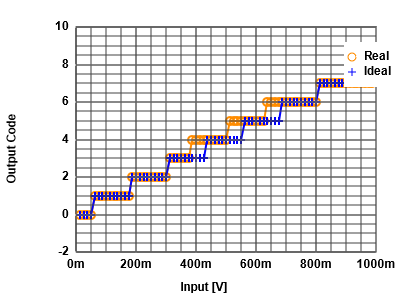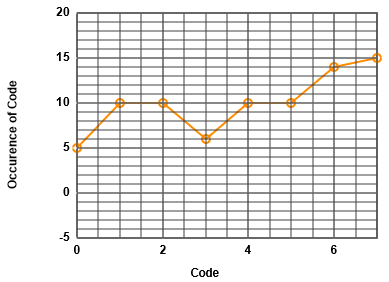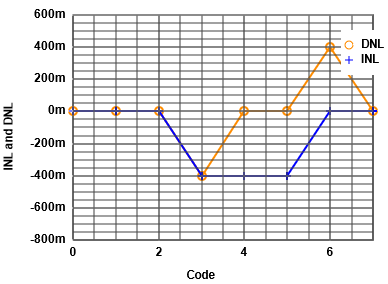Histogramm test example with noise

Number of Bits: 3
Noise: 0.02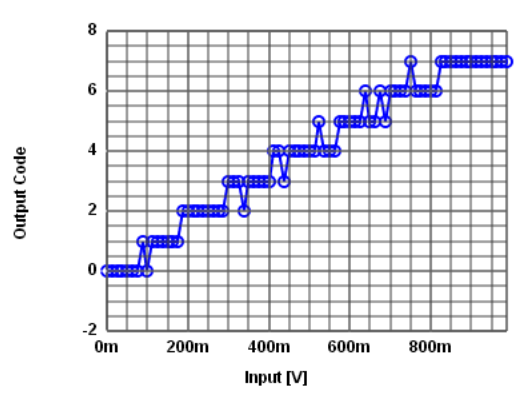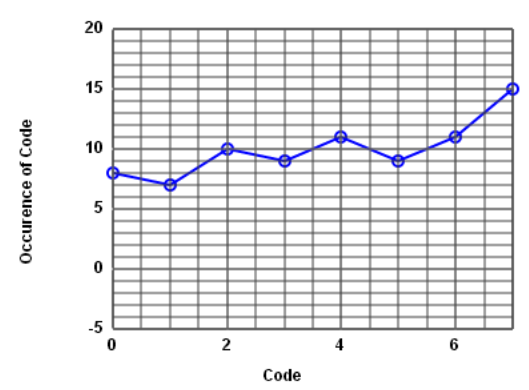Histogramm test with sine input signal

 It is difficult to build an analog linear ramp generator. A higher resolution DAC can be used. A sine input signal can be used. A high quality filter eliminates harmonics. Occurence will not be flat, but can be calculated in advance to normalize occurence to calculate INL and DNL. Occurence has a bathtub shape. At top and bottom highest occcurence can be seen, since the sine signal is flat. In the center low occurence can be seen due to the high slope of the sine signal. It is difficult to match the sine signal exactly to the input range of the ADC.
This example shows a 4 Bit test using 400 measurement points.

Histogramm sine signal

 How many samples M do you need of a sine input signal for a N Bit data converter? There will be more samples available at the top and bottom and less in the center. Signal: $V(t) = 2^{N-1} \cdot LSB \cdot sin \left( \omega t \right)$ Amplitude is: $2^{N-1} \cdot LSB$ $V'(t) = 2^{N-1} \cdot LSB \cdot \omega \cdot cos \left( \omega t \right)$ Maximum change in signal after dt should be smaller than LSB: $2^{N-1} \cdot LSB \cdot \omega \cdot dt \lt LSB$ with $\omega = \frac{ 2 \pi}{M dt}$ $M \gt 2 \cdot \pi \cdot 2^{N-1} \approx 3 \cdot 2^N$ Having 16 times 2N samples gives an accuracy for INL and DNL of $\frac{3}{16} \approx 0.2$. Having 8 times 2N samples gives an accuracy for INL and DNL of $\frac{3}{8} \approx 0.375$.

Histogramm sine signal accuracy

Error in sine signal estimate

Make sure integer number of periods are used.
Y = round((2^(n-1) - 0.5) + (2^(n-1) - 0.5) * sin(x*11/gr*2PI)) ;
Y = round((2^(n-1) - 0.9) + ((2^(n-1) - 0.5) * sin(x*11/gr*2PI)) ;
Y = round((2^(n-1) - 0.5) + ((2^(n-1) - 0.1) * sin(x*11/gr*2PI)) ;
Bits: 5
Range: 0..31
1) Average shift: - 0.4LSB
2) Amplitude increased: + 0.4LSB
A slight error in estimation of number of periods, amplitude and offset of sine signal can lead to codes in the limited range of ADC having big INL and DNL errors.
Fit a curve with minimum error.

Histogramm sine signal practical test setup

A real Histogram implementation needs RAM:
• Sine signal buffer: $2^{N} \cdot 4 \cdot N$
• Error correction lookup DAC: $2^{N} \cdot N$
• Error correction lookup ADC: $2^{N} \cdot N$
• Output buffer: $2^{N} \cdot 16 \cdot N$
In total $2^{N} \cdot 22 \cdot N$ bits RAM.

A NEXYS3 Spartan 6 xc6slx16 FPGA board has 32 Blocks of 18Kbit block RAM.
This allows a 10 Bit converter to be implemented in FPGA: $2^{10} \cdot 22 \cdot 10 \approx 23$ blocks.

NEXYS3 external 16MByte Cellular RAM: 16 Bit asynchronous 70ns (14 MHz), synchronous 80 MHz.
Read sine from lookup in 83 ns, read SAR output and get lookup corrected output, store corrected output.
250ns cycle time: 83ns each, 4 MHz
16 Bit: 65k * 22 * 16 = 1365kBit * 16 = 2.8 MByte
18 Bit: 256k * 22 * 18 = 12 MByte
Up to 18 Bit at 4 MHz are possible with external RAM.

When is a CORDIC sine calculation better than a lookup table?

Characterization System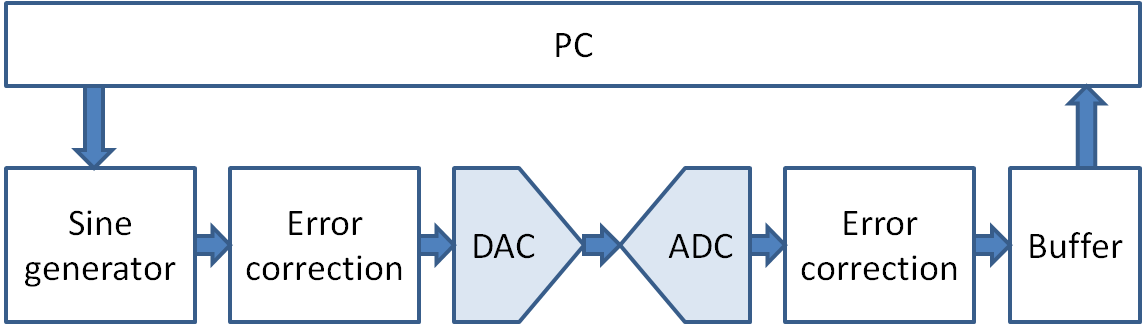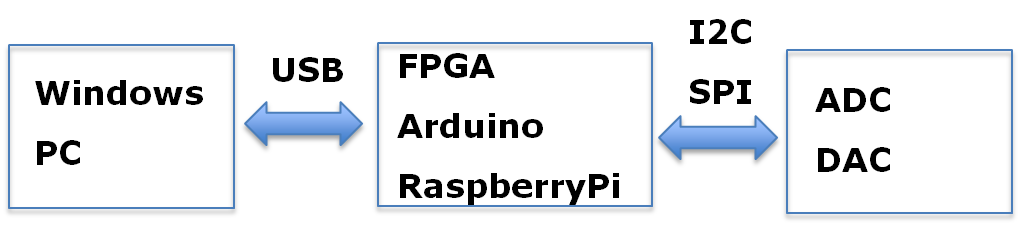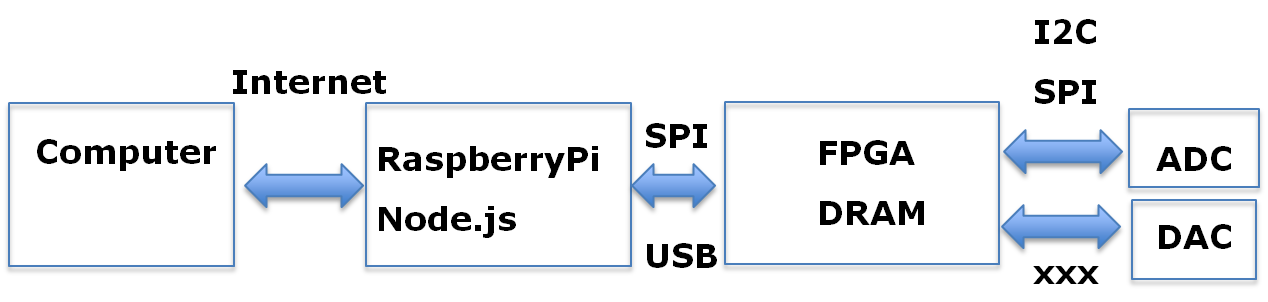IEEE Data converter Measurement

IEEE standards 1241 and 1057  
describe the most effective ADC testing procedures to apply for evaluating figures of merit of such devices. Methodology usually employed are based on the analysis of data generated at the ADC output when a spectrally pure single- or dual-tone is applied to the input of the device under test (DUT).

Practical Histogramm Testing

• Histogramm test assumes monotonicity.
• Testing many devices gives statistical and systematic error.
• Always look at DNL and INL.
• Even with a low DNL INL can be quiet high by accumulating DNL error.

Dynamic testing
04 Spectral test

References:

 M. V. Bossche, J. Schoukens, and J. Renneboog, “Dynamic Testing and Diagnostics of A/D Converters,” IEEE Transactions on Circuits and Systems, vol. CAS-33, no. 8, Aug. 1986.
 IEEE Standard 1057
 IEEE Standard 1241
IEEE Std 1241